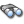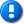home | career | drupal | java | mac | mysql | perl | scala | uml | unix

# Java example source code file (OLSMultipleLinearRegression.java)

This example Java source code file (OLSMultipleLinearRegression.java) is included in the alvinalexander.com "Java Source Code Warehouse" project. The intent of this project is to help you "Learn Java by Example" TM.

Learn more about this Java project at its project page.

## Java - Java tags/keywords

abstractmultiplelinearregression, array2drowrealmatrix, mathillegalargumentexception, olsmultiplelinearregression, override, qrdecomposition, raug, realmatrix, realvector, rinv

## The OLSMultipleLinearRegression.java Java example source code

```/*
* Licensed to the Apache Software Foundation (ASF) under one or more
* contributor license agreements.  See the NOTICE file distributed with
* this work for additional information regarding copyright ownership.
* The ASF licenses this file to You under the Apache License, Version 2.0
* (the "License"); you may not use this file except in compliance with
* the License.  You may obtain a copy of the License at
*
*      http://www.apache.org/licenses/LICENSE-2.0
*
* Unless required by applicable law or agreed to in writing, software
* distributed under the License is distributed on an "AS IS" BASIS,
* WITHOUT WARRANTIES OR CONDITIONS OF ANY KIND, either express or implied.
* See the License for the specific language governing permissions and
* limitations under the License.
*/
package org.apache.commons.math3.stat.regression;

import org.apache.commons.math3.exception.MathIllegalArgumentException;
import org.apache.commons.math3.linear.Array2DRowRealMatrix;
import org.apache.commons.math3.linear.LUDecomposition;
import org.apache.commons.math3.linear.QRDecomposition;
import org.apache.commons.math3.linear.RealMatrix;
import org.apache.commons.math3.linear.RealVector;
import org.apache.commons.math3.stat.StatUtils;
import org.apache.commons.math3.stat.descriptive.moment.SecondMoment;

/**
* <p>Implements ordinary least squares (OLS) to estimate the parameters of a
* multiple linear regression model.</p>
*
* <p>The regression coefficients, `b`, satisfy the normal equations:
* <pre>` XT X b = XT y ````

* * <p>To solve the normal equations, this implementation uses QR decomposition * of the <code>X matrix. (See {@link QRDecomposition} for details on the * decomposition algorithm.) The <code>X matrix, also known as the design matrix, * has rows corresponding to sample observations and columns corresponding to independent * variables. When the model is estimated using an intercept term (i.e. when * {@link #isNoIntercept() isNoIntercept} is false as it is by default), the <code>X * matrix includes an initial column identically equal to 1. We solve the normal equations * as follows: * <pre>``` XTX b = XT y * (QR)<sup>T (QR) b = (QR)Ty * R<sup>T (QTQ) R b = RT QT y * R<sup>T R b = RT QT y * (R<sup>T)-1 RT R b = (RT)-1 RT QT y * R b = Q<sup>T y ```

* * <p>Given `Q` and `R`, the last equation is solved by back-substitution.

* * @since 2.0 */ public class OLSMultipleLinearRegression extends AbstractMultipleLinearRegression { /** Cached QR decomposition of X matrix */ private QRDecomposition qr = null; /** Singularity threshold for QR decomposition */ private final double threshold; /** * Create an empty OLSMultipleLinearRegression instance. */ public OLSMultipleLinearRegression() { this(0d); } /** * Create an empty OLSMultipleLinearRegression instance, using the given * singularity threshold for the QR decomposition. * * @param threshold the singularity threshold * @since 3.3 */ public OLSMultipleLinearRegression(final double threshold) { this.threshold = threshold; } /** * Loads model x and y sample data, overriding any previous sample. * * Computes and caches QR decomposition of the X matrix. * @param y the [n,1] array representing the y sample * @param x the [n,k] array representing the x sample * @throws MathIllegalArgumentException if the x and y array data are not * compatible for the regression */ public void newSampleData(double[] y, double[][] x) throws MathIllegalArgumentException { validateSampleData(x, y); newYSampleData(y); newXSampleData(x); } /** * {@inheritDoc} * <p>This implementation computes and caches the QR decomposition of the X matrix.

*/ @Override public void newSampleData(double[] data, int nobs, int nvars) { super.newSampleData(data, nobs, nvars); qr = new QRDecomposition(getX(), threshold); } /** * <p>Compute the "hat" matrix. * </p> * <p>The hat matrix is defined in terms of the design matrix X * by X(X<sup>TX)-1XT * </p> * <p>The implementation here uses the QR decomposition to compute the * hat matrix as Q I<sub>pQT where Ip is the * p-dimensional identity matrix augmented by 0's. This computational * formula is from "The Hat Matrix in Regression and ANOVA", * David C. Hoaglin and Roy E. Welsch, * <i>The American Statistician, Vol. 32, No. 1 (Feb., 1978), pp. 17-22. * </p> * <p>Data for the model must have been successfully loaded using one of * the {@code newSampleData} methods before invoking this method; otherwise * a {@code NullPointerException} will be thrown.</p> * * @return the hat matrix * @throws NullPointerException unless method {@code newSampleData} has been * called beforehand. */ public RealMatrix calculateHat() { // Create augmented identity matrix RealMatrix Q = qr.getQ(); final int p = qr.getR().getColumnDimension(); final int n = Q.getColumnDimension(); // No try-catch or advertised NotStrictlyPositiveException - NPE above if n < 3 Array2DRowRealMatrix augI = new Array2DRowRealMatrix(n, n); double[][] augIData = augI.getDataRef(); for (int i = 0; i < n; i++) { for (int j =0; j < n; j++) { if (i == j && i < p) { augIData[i][j] = 1d; } else { augIData[i][j] = 0d; } } } // Compute and return Hat matrix // No DME advertised - args valid if we get here return Q.multiply(augI).multiply(Q.transpose()); } /** * <p>Returns the sum of squared deviations of Y from its mean.

* * <p>If the model has no intercept term, `0` is used for the * mean of Y - i.e., what is returned is the sum of the squared Y values.</p> * * <p>The value returned by this method is the SSTO value used in * the {@link #calculateRSquared() R-squared} computation.</p> * * @return SSTO - the total sum of squares * @throws NullPointerException if the sample has not been set * @see #isNoIntercept() * @since 2.2 */ public double calculateTotalSumOfSquares() { if (isNoIntercept()) { return StatUtils.sumSq(getY().toArray()); } else { return new SecondMoment().evaluate(getY().toArray()); } } /** * Returns the sum of squared residuals. * * @return residual sum of squares * @since 2.2 * @throws org.apache.commons.math3.linear.SingularMatrixException if the design matrix is singular * @throws NullPointerException if the data for the model have not been loaded */ public double calculateResidualSumOfSquares() { final RealVector residuals = calculateResiduals(); // No advertised DME, args are valid return residuals.dotProduct(residuals); } /** * Returns the R-Squared statistic, defined by the formula <pre> * R<sup>2 = 1 - SSR / SSTO * </pre> * where SSR is the {@link #calculateResidualSumOfSquares() sum of squared residuals} * and SSTO is the {@link #calculateTotalSumOfSquares() total sum of squares} * * <p>If there is no variance in y, i.e., SSTO = 0, NaN is returned.

* * @return R-square statistic * @throws NullPointerException if the sample has not been set * @throws org.apache.commons.math3.linear.SingularMatrixException if the design matrix is singular * @since 2.2 */ public double calculateRSquared() { return 1 - calculateResidualSumOfSquares() / calculateTotalSumOfSquares(); } /** * <p>Returns the adjusted R-squared statistic, defined by the formula
```     * R<sup>2adj = 1 - [SSR (n - 1)] / [SSTO (n - p)]
* </pre>
* where SSR is the {@link #calculateResidualSumOfSquares() sum of squared residuals},
* SSTO is the {@link #calculateTotalSumOfSquares() total sum of squares}, n is the number
* of observations and p is the number of parameters estimated (including the intercept).</p>
*
* <p>If the regression is estimated without an intercept term, what is returned is      * <code> 1 - (1 - {@link #calculateRSquared()}) * (n / (n - p))
* </pre>
*
* <p>If there is no variance in y, i.e., SSTO = 0, NaN is returned.
*
* @return adjusted R-Squared statistic
* @throws NullPointerException if the sample has not been set
* @throws org.apache.commons.math3.linear.SingularMatrixException if the design matrix is singular
* @see #isNoIntercept()
* @since 2.2
*/
public double calculateAdjustedRSquared() {
final double n = getX().getRowDimension();
if (isNoIntercept()) {
return 1 - (1 - calculateRSquared()) * (n / (n - getX().getColumnDimension()));
} else {
return 1 - (calculateResidualSumOfSquares() * (n - 1)) /
(calculateTotalSumOfSquares() * (n - getX().getColumnDimension()));
}
}

/**
* {@inheritDoc}
* <p>This implementation computes and caches the QR decomposition of the X matrix
* once it is successfully loaded.</p>
*/
@Override
protected void newXSampleData(double[][] x) {
super.newXSampleData(x);
qr = new QRDecomposition(getX(), threshold);
}

/**
* Calculates the regression coefficients using OLS.
*
* <p>Data for the model must have been successfully loaded using one of
* the {@code newSampleData} methods before invoking this method; otherwise
* a {@code NullPointerException} will be thrown.</p>
*
* @return beta
* @throws org.apache.commons.math3.linear.SingularMatrixException if the design matrix is singular
* @throws NullPointerException if the data for the model have not been loaded
*/
@Override
protected RealVector calculateBeta() {
return qr.getSolver().solve(getY());
}

/**
* <p>Calculates the variance-covariance matrix of the regression parameters.
* </p>
* <p>Var(b) = (XTX)-1
* </p>
* <p>Uses QR decomposition to reduce (XTX)-1
* to (R<sup>TR)-1, with only the top p rows of
* R included, where p = the length of the beta vector.</p>
*
* <p>Data for the model must have been successfully loaded using one of
* the {@code newSampleData} methods before invoking this method; otherwise
* a {@code NullPointerException} will be thrown.</p>
*
* @return The beta variance-covariance matrix
* @throws org.apache.commons.math3.linear.SingularMatrixException if the design matrix is singular
* @throws NullPointerException if the data for the model have not been loaded
*/
@Override
protected RealMatrix calculateBetaVariance() {
int p = getX().getColumnDimension();
RealMatrix Raug = qr.getR().getSubMatrix(0, p - 1 , 0, p - 1);
RealMatrix Rinv = new LUDecomposition(Raug).getSolver().getInverse();
return Rinv.multiply(Rinv.transpose());
}

}

Other Java examples (source code examples)
Here is a short list of links related to this Java OLSMultipleLinearRegression.java source code file:The search pageOther Java source code examples at this package levelClick here to learn more about this project

```

Copyright 1998-2021 Alvin Alexander, alvinalexander.com
All Rights Reserved.

A percentage of advertising revenue from
pages under the /java/jwarehouse URI on this website is
paid back to open source projects.